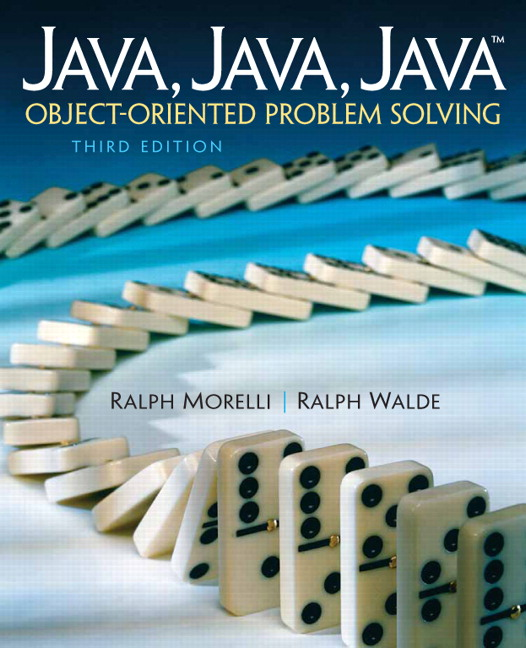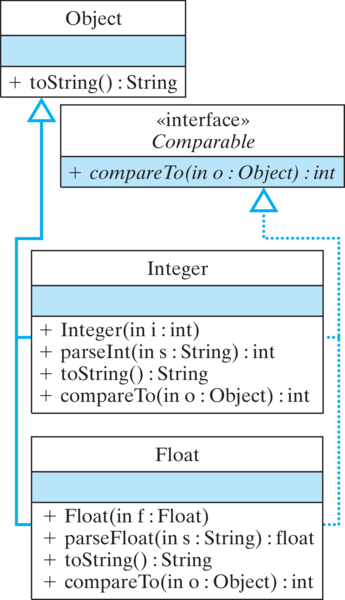# Java, Java, Java: Object-Oriented Problem Solving, 2022E

## Section9.9OBJECT-ORIENTED DESIGN: Polymorphic Sorting (Optional)

One limitation of the sort routines developed so far is that they only work on one particular type of data. If you’ve written an insertion sort to sort ints, you can’t use it to sort doubles.
What would be far more desirable is a polymorphic sort method—that is, one method that could sort any kind of data. This is easily done by making use of Java wrapper classes, such as Integer and Double, together with the java.lang.Comparable interface, which is specially designed for this purpose.

### Subsection9.9.1The Comparable Interface

The java.lang.Comparable interface consists of the compareTo() method:
public abstract interface Comparable {
public int compareTo (Object o);  // Abstract method}

By implementing compareTo(), a class can impose an order on its objects. The Comparable interface is implemented by all of Java’s wrapper classes — that is, by Integer, Double, Float, Long, and so on (Fig. 9.24).Figure 9.9.1. : Java wrapper classes, such as Integer and Float, implement the Comparable interface.
As we saw in Chapter 8, Java interfaces allow us to create a form of multiple inheritance. For example, as Figure 9.9.1 shows, an Integer is both an Object and a Comparable. One implication of this is that an Integer can be used in any method that takes either an Object parameter or a Comparable parameter.
The compareTo() method takes an Object parameter and returns an int. It is meant to be invoked as o1.compareTo(o2), where o1 and o2 are objects of the same type. Classes that implement compareTo() must abide by the following rules for its return value:
if (o1 < o2)    then o1.compareTo(o2) < 0
if (o1.equals(o2)) then o1.compareTo(o2) == 0
if (o1 > o2)       then o1.compareTo(o2) > 0

In other words, if o1 < o2, then o1.compareTo(o2) will return a negative integer. If o1 > o2, then o1.compareTo(o2) will return a positive integer. And if o1 and o2 are equal, then o1.compareTo(o2) will return 0.

### Subsection9.9.2Sorting Comparables

For a class that implements Comparable, we can use the compareTo() method to help sort its elements. For example, the following revised version of insertionSort() method can be used to sort any array of Comparable objects—that is, any array of objects whose class implements Comparable for any type T.
  public  <T extends Comparable<T>> void sort(T[] arr) {
T temp;      // Temporary variable for insertion

for (int k = 1; k < arr.length; k++)  {
temp = arr[k];    // Remove it from array
int i;
for (i = k-1; i >= 0 && arr[i].compareTo(temp) > 0; i--)
arr[i+1] = arr[i];   // Move it right by one
arr[i+1] = temp;        // Insert the element
}
} // sort()

In this version, the parameter is an array of any Object type T. Thus, we can pass it an array of Integer or Float, and so on. Then, to compare elements of a Comparable array, we use the compareTo() method:
for (i = k-1; i >= 0 && arr[i].compareTo(temp) > 0; i--)

Note that our algorithm no longer refers to ints, as in the original insertion sort. Indeed, it doesn’t mention the specific type—Integer, Float, or whatever — of the objects that it is sorting. It refers only to Comparable s. Therefore, we can use this method to sort any type of object, as long as the object’s class implements the Comparable interface. Thus, by using Comparable, we have a more general insertionSort() method, one that can sort any one-dimensional array of Comparable s.
The TestSort class (Figure 9.9.2 and Listing 9.9.3) provides an example of how to use the polymorphic sort() method.
It contains three methods: The sort() method that we just described; a polymorphic print() method, which can be used to print the values of any array of Comparable; and a main() method. The main() method creates arrays of Integer and Float and then uses the polymorphic sort() method to sort them. Note how the print() method uses the polymorphic toString() method to print the elements of a Comparable array.
This example of polymorphic sorting illustrates once again the great power of inheritance and polymorphism in object-oriented programming. The Integer and Float classes use class inheritance to inherit features from the Object class, and they use interface implementation to inherit the compareTo() method from the Comparable class. By implementing versions of the toString() and compareTo() methods that are appropriate for these wrapper classes, Java makes it easier to use Integer and Float objects in a variety of contexts.
Taken together, inheritance and polymorphism enable us to design very general and extensible algorithms. In this example, we defined a sort() method that can sort an array containing any kind of object as long as the object implements the Comparable interface.

### Subsection9.9.3The java.util.Arrays.sort() Method

While sorting algorithms provide a good way to introduce the concepts of array processing, real-world programmers never write their own sort algorithms. Instead they use library methods, which have been written and optimized by programming experts. Moreover, library sort routines use sort algorithms that are much more efficient than the ones we’ve discussed.
The java.util.Arrays class contains a polymorphic sort method that is very simple to use. For example, here’s how we would use it to sort the two arrays declared in the TestSort program:
java.util.Arrays.sort(iArr);
// or if you: import java.util.*;
Arrays.sort(fArr);

That’s all there is to it! Obviously, learning how to use Java’s class and method library, saves real-word programmers lots of effort. Try it below!

#### Activity9.9.1.

Run the code below a couple times to populate the array with random numbers and see the result of the Arrays.sort() method.

#### ExercisesSelf-Study Exercises

##### 1.Polymorphic Sort.
This exercise has three parts:
1. Add a definition of a compareTo() method to the LetterFreq class so that it implements the Comparable interface. The method should define one object to be less than another object if its freq instance variable is less.
2. Add a definition of a sort() method that can be added to the definition of the AnalyzeFreq class. Make it so the array in the class can be sorted into ascending order using the ordering of LetterFreq defined in the previous exercise. Use the java.util.Arrays.sort() method.
3. Rewrite the main() of the AnalyzeFreq class to make use of the sort() method of the previous exercise.